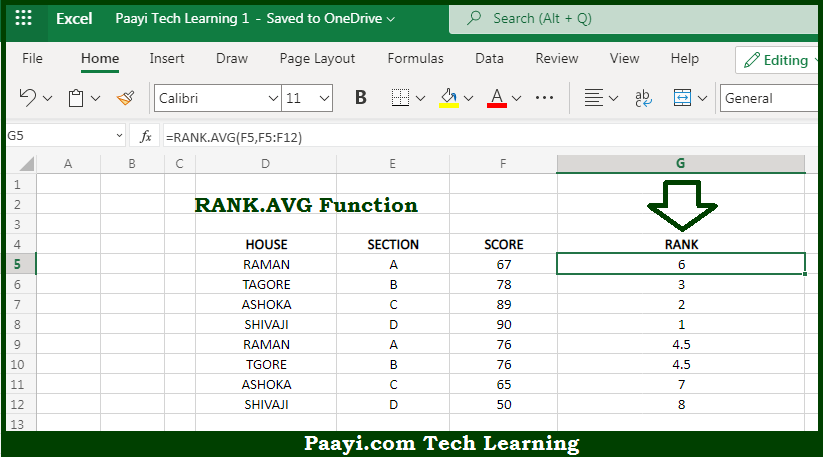# Learn How to Use Microsoft Excel RANK.AVG Function

Written by | 0 Comments | 618 Views

In this article, you will learn how to use the Microsoft Excel RANK.AVG function and its prime function in Microsoft Excel. You will also get to know the Microsoft Excel RANK.AVG function return value and syntax with the help of some examples.

Microsoft Excel RANK.AVG Function

The main purpose of the Microsoft Excel RANK.AVG function is to rank a number against a range of numbers. That implies, with the help of RANK.AVG function you can able to return the rank of a number against a list of other numeric values. It should be noted that when values contain duplicates, the RANK.AVG function will assign an average rank to each set of duplicates. So, with the help of RANK.AVG function, you can able to rank a number against the range of numbers.

Return Value of RANK.AVG Function

The return value will be the number indicating rank.

Syntax of RANK.AVG Function

=RANK.AVG(number, ref, [order])

Where the arguments:

• number: This is the number to rank.
• ref: This is the range or array that contains the numbers to rank against.
• order: Whether to rank in ascending or descending order, default is zero (optional).

## How to Use Microsoft Excel RANK.AVG Function?So we know that, Microsoft Excel RANK.AVG function you can able to rank a number against the range of numbers. That implies, with the help of RANK.AVG function you can able to return the rank of a number against a list of other numeric values. It should be noted that when values contain duplicates, the RANK.AVG function will assign an average rank to each set of duplicates. So, with the help of RANK.AVG function, you can able to rank a number against the range of numbers.